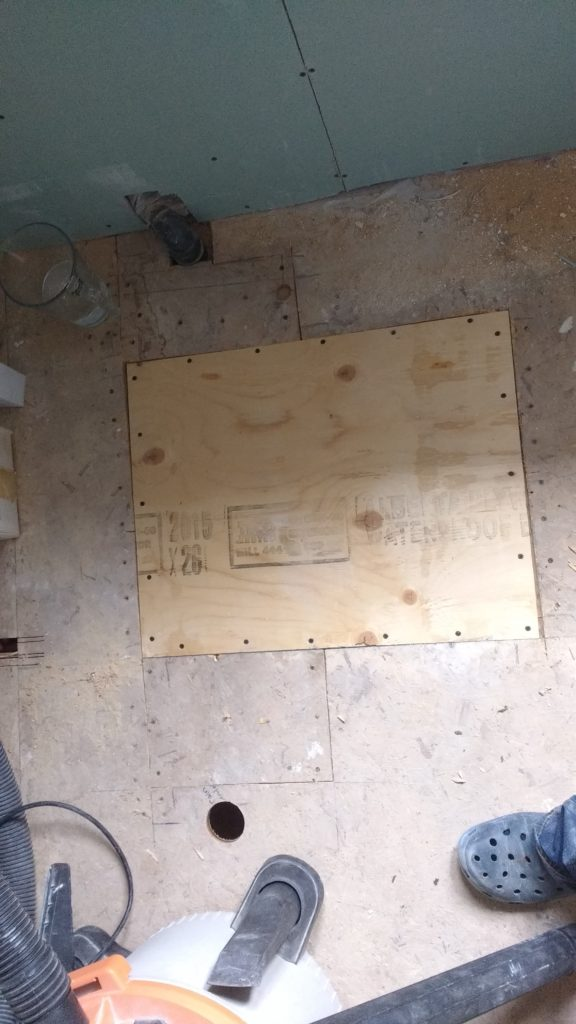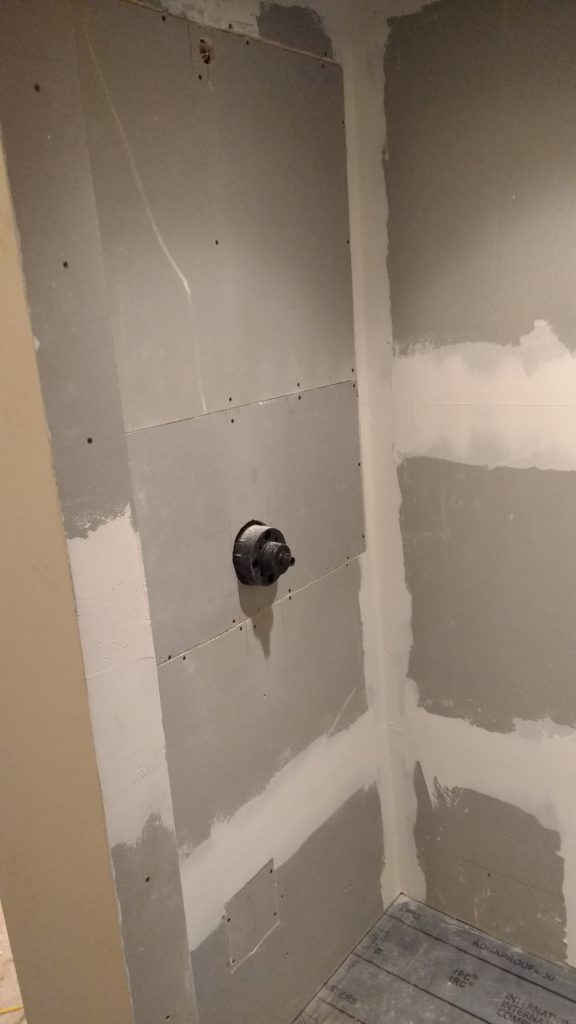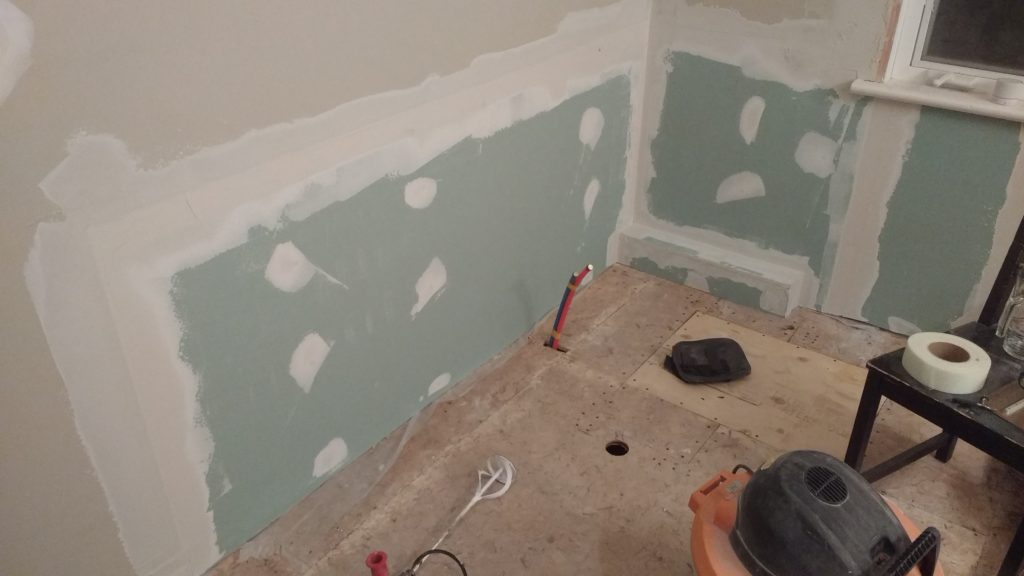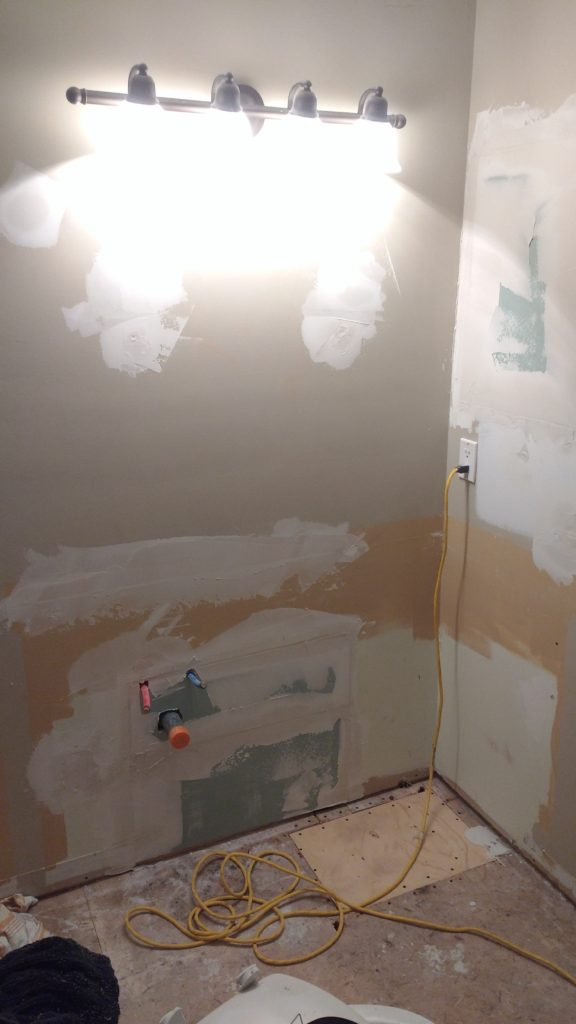## Some progress prepping the bathroom reno.

We are finally renovating our master bathroom. We’ve hired the guys from Markham Tile to do the plumbing and tile work (especially in the shower, which I was afraid to do). They don’t do the drywall part of the job, and left me some floor repairs as well. Here’s where the plumbing was done for the new tub:In this picture, I’ve started installing some 2×4 “strapping” to support the replacement floor boards. I also ended expanding the holes a bit so that I could fit in pieces that used the joists to hold things up. Here’s things with all the puzzle pieces installed:Over where the sink will be, I also had a mess to deal with:I ended up expanding that hole a bit too, mostly to make a rectangular hole that would be easier to patch. I also installed some 2×4 “strapping” in this hole for extra support:The final installed floor patch looks like:With flooring in place, after a lot of tidy up, and installation of a small bump-out around some pipe that couldn’t be moved, I was ready to start throwing around some mud. Here’s the pre-mud view:and by the sink:This view shows where the medicine cabinet used to be. We removed it so that the shower head could be in a less awkward spot (it’s now centered in the shower). Unfortunately, moving the shower head and control left me some shower board repair to do. Here’s a pre-mud view of the shower where I’m doing the repair:We opted not to install the “toe tester” in this shower rebuild since we were adjusting the plumbing anyways.

Here’s the bathtub area after an initial rough layup of mud:and the shower board repair:and a really badly lit shot of the sink area:Tomorrow I lay up a bit more mud.

## What fun or important formulas would you put on a shirt?

June 2, 2018 Just for fun ,

Lance got a custom made glow in the dark “math, physics, and chemistry” shirt for his birthday a year or two ago (It was Sofia’s idea, she bought the materials, and I got to play.)He is clearly unimpressed with the photo op, but I wanted to share this shirt, which includes some of the what I thought were a number of the most interesting or important relationships

• Gamma function, which generalizes factorial to non-integer values.
\begin{equation}\label{eqn:formulas:20}
\Gamma(z + 1) = \int_0^\infty t^z e^{-t} dt.
\end{equation}
This formula satisfies $$n! = \Gamma(n + 1)$$.

• Euler’s:
\begin{equation}\label{eqn:formulas:40}
e^{n i \theta} = \lr{ \cos\theta + i \sin\theta }^n.
\end{equation}

• Schr\”{o}dinger’s equation
\begin{equation}\label{eqn:formulas:60}
i \, \hbar \PD{t}{} \ket{\psi} = H \ket{\psi}.
\end{equation}

• Taylor series
\begin{equation}\label{eqn:formulas:80}
f(x) = \sum_{k = 0}^\infty \frac{f^{(k)}(0)}{k!} x^k.
\end{equation}

• Euler-Lagrange equations:
\begin{equation}\label{eqn:formulas:100}
\PD{x_i}{\LL} = \frac{d}{dt} \PD{\dot{x}_i}{\LL}.
\end{equation}
These formulas can be used to express most dynamics relations. You can think of them as basically being the consequence that physical laws are either inherently greedy or lazy.

• Stokes’ theorem, in its geoemetric algebra form
\begin{equation}\label{eqn:formulas:120}
\oint_{\partial V} d^n \Bx \cdot T = \int_V d^n \Bx \cdot \lr{ \spacegrad \cdot T }.
\end{equation}

• Quantum commutator relationships between position and momentum
\begin{equation}\label{eqn:formulas:140}
\antisymmetric{X}{P} = i \, \hbar.
\end{equation}

• Fourier transform
\begin{equation}\label{eqn:formulas:160}
\tilde{f}(\omega) = \int_{-\infty}^\infty f(t) e^{-i \omega t} dt.
\end{equation}

• Vector product
\begin{equation}\label{eqn:formulas:180}
\Ba \Bb = \Ba \cdot \Bb + \Ba \wedge \Bb.
\end{equation}
In geometric algebra the vector product has a dot and wedge product split. In \R{3} you can write this as
$$\Ba \Bb = \Ba \cdot \Bb + I (\Ba \cross \Bb)$$, where $$I = \Be_1 \Be_2 \Be_3$$.

• Relativisitic energy (Einstein’s)
\begin{equation}\label{eqn:formulas:200}
E = \frac{m c^2}{\sqrt{1 – (\Bv/c)^2}}.
\end{equation}

• Cauchy contour integral relationships for the nth derivative
\begin{equation}\label{eqn:formulas:220}
f^{(n)}(s) = \frac{n!}{2 \pi i} \int_C dz \frac{f(z)}{(z-s)^{n+1}}
\end{equation}

• Maxwell’s equations
\begin{equation}\label{eqn:formulas:240}
\begin{aligned}
\partial_\mu F^{\mu\nu} &= J^\nu \\
\epsilon^{\mu \nu \rho \sigma} \partial_\nu F_{\rho \sigma} &= 0.
\end{aligned}
\end{equation}
I’m partial to the geometric algebra form of Maxwell’s equations $$\grad F = J$$, but that wouldn’t have looked as good on the shirt.

• Dirac equation
\begin{equation}\label{eqn:formulas:260}
0 = \lr{ i \gamma^\mu \partial_\mu – m } \psi.
\end{equation}

If you had to pick a set of important formulas on a tee-shirt, which ones would you pick?